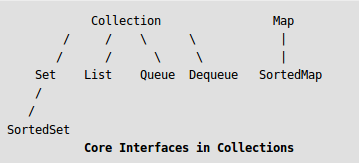# Program to Convert List to Map in Java

• Difficulty Level : Medium
• Last Updated : 19 Jun, 2019

The List is a child interface of Collection. It is an ordered collection of objects in which duplicate values can be stored. Since List preserves the insertion order, it allows positional access and insertion of elements. List Interface is implemented by ArrayList, LinkedList, Vector and Stack classes.

The java.util.Map interface represents a mapping between a key and a value. The Map interface is not a subtype of the Collection interface. Therefore it behaves a bit different from the rest of the collection types.Example:

```Input: List : [1="1", 2="2", 3="3"]
Output: Map : {1=1, 2=2, 3=3}

Input: List : [1="Geeks", 2="for", 3="Geeks"]
Output: Map : {1=Geeks, 2=for, 3=Geeks}
```

Below are various ways to convert List to Map in Java. For this, it is assumed that each element of the List has an identifier which will be used as a key in the resulting Map.

1. Using by object of list:

Approach:

1. Get the List to be converted into Map
2. Create an empty Map
3. Iterate through the items in the list and add each of them into the Map.
4. Return the formed Map

 `// Java program for list convert in map``// with the help of Object method`` ` `import` `java.util.ArrayList;``import` `java.util.List;``import` `java.util.Map;``import` `java.util.HashMap;`` ` `// create a list``class` `Student {`` ` `    ``// id will act as Key``    ``private` `Integer id;`` ` `    ``// name will act as value``    ``private` `String name;`` ` `    ``// create curstuctor for reference``    ``public` `Student(Integer id, String name)``    ``{`` ` `        ``// assign the value of id and name``        ``this``.id = id;``        ``this``.name = name;``    ``}`` ` `    ``// return private variable id``    ``public` `Integer getId()``    ``{``        ``return` `id;``    ``}`` ` `    ``// return private variable name``    ``public` `String getName()``    ``{``        ``return` `name;``    ``}``}`` ` `// main class and method``public` `class` `GFG {`` ` `    ``// main Driver``    ``public` `static` `void` `main(String[] args)``    ``{`` ` `        ``// create a list``        ``List``            ``lt = ``new` `ArrayList();`` ` `        ``// add the member of list``        ``lt.add(``new` `Student(``1``, ``"Geeks"``));``        ``lt.add(``new` `Student(``2``, ``"For"``));``        ``lt.add(``new` `Student(``3``, ``"Geeks"``));`` ` `        ``// create map with the help of``        ``// Object (stu) method``        ``// create object of Map class``        ``Map map = ``new` `HashMap<>();`` ` `        ``// put every value list to Map``        ``for` `(Student stu : lt) {``            ``map.put(stu.getId(), stu.getName());``        ``}`` ` `        ``// print map``        ``System.out.println(``"Map  : "` `+ map);``    ``}``}`

Output:

```Map  : {1=Geeks, 2=For, 3=Geeks}
```
2. Using Collectors.toMap() method: This method includes creation of a list of the student objects, and uses Collectors.toMap() to convert it into a Map.
Approach:

1. Get the List to be converted into Map
2. Convert the List into stream using List.stream() method
3. Create map with the help of Collectors.toMap() method
4. Collect the formed Map using stream.collect() method
5. Return the formed Map

 `// Java program for list convert  in map``// with the help of Collectors.toMap() method`` ` `import` `java.util.ArrayList;``import` `java.util.LinkedHashMap;``import` `java.util.List;``import` `java.util.stream.Collectors;`` ` `// create a list``class` `Student {`` ` `    ``// id will act as Key``    ``private` `Integer id;`` ` `    ``// name will act as value``    ``private` `String name;`` ` `    ``// create curstuctor for reference``    ``public` `Student(Integer id, String name)``    ``{`` ` `        ``// assign the value of id and name``        ``this``.id = id;``        ``this``.name = name;``    ``}`` ` `    ``// return private variable id``    ``public` `Integer getId()``    ``{``        ``return` `id;``    ``}`` ` `    ``// return private variable name``    ``public` `String getName()``    ``{``        ``return` `name;``    ``}``}`` ` `// main class and method``public` `class` `GFG {`` ` `    ``// main Driver``    ``public` `static` `void` `main(String[] args)``    ``{`` ` `        ``// create a list``        ``List lt = ``new` `ArrayList<>();`` ` `        ``// add the member of list``        ``lt.add(``new` `Student(``1``, ``"Geeks"``));``        ``lt.add(``new` `Student(``2``, ``"For"``));``        ``lt.add(``new` `Student(``3``, ``"Geeks"``));`` ` `        ``// create map with the help of``        ``// Collectors.toMap() method``        ``LinkedHashMap``            ``map = lt.stream()``                      ``.collect(``                          ``Collectors``                              ``.toMap(``                                  ``Student::getId,``                                  ``Student::getName,``                                  ``(x, y)``                                      ``-> x + ``", "` `+ y,``                                  ``LinkedHashMap::``new``));`` ` `        ``// print map``        ``map.forEach(``            ``(x, y) -> System.out.println(x + ``"="` `+ y));``    ``}``}`

Output:

```1=Geeks
2=For
3=Geeks
```
3. Creating MultiMap using Collectors.groupingBy():

Approach:

1. Get the List to be converted into Map
2. Convert the List into stream using List.stream() method
3. Create map with the help of Collectors.groupingBy() method
4. Collect the formed Map using stream.collect() method
5. Return the formed Map

 `// Java program for list convert  in map``// with the help of Collectors.groupingBy() method`` ` `import` `java.util.*;``import` `java.util.stream.Collectors;`` ` `// create a list``class` `Student {`` ` `    ``// id will act as Key``    ``private` `Integer id;`` ` `    ``// name will act as value``    ``private` `String name;`` ` `    ``// create curstuctor for reference``    ``public` `Student(Integer id, String name)``    ``{`` ` `        ``// assign the value of id and name``        ``this``.id = id;``        ``this``.name = name;``    ``}`` ` `    ``// return private variable id``    ``public` `Integer getId()``    ``{``        ``return` `id;``    ``}`` ` `    ``// return private variable name``    ``public` `String getName()``    ``{``        ``return` `name;``    ``}``}`` ` `// main class and method``public` `class` `GFG {`` ` `    ``// main Driver``    ``public` `static` `void` `main(String[] args)``    ``{`` ` `        ``// create a list``        ``List lt = ``new` `ArrayList();`` ` `        ``// add the member of list``        ``lt.add(``new` `Student(``1``, ``"Geeks"``));``        ``lt.add(``new` `Student(``1``, ``"For"``));``        ``lt.add(``new` `Student(``2``, ``"Geeks"``));``        ``lt.add(``new` `Student(``2``, ``"GeeksForGeeks"``));`` ` `        ``// create map with the help of``        ``// Object (stu) method``        ``// create object of Multi Map class`` ` `        ``// create multimap and store the value of list``        ``Map >``            ``multimap = lt``                           ``.stream()``                           ``.collect(``                               ``Collectors``                                   ``.groupingBy(``                                       ``Student::getId,``                                       ``Collectors``                                           ``.mapping(``                                               ``Student::getName,``                                               ``Collectors``                                                   ``.toList())));`` ` `        ``// print the multiMap``        ``System.out.println(``"MultiMap = "` `+ multimap);``    ``}``}`

Output:

```MultiMap = {1=[Geeks, For], 2=[Geeks, GeeksForGeeks]}
```

My Personal Notes arrow_drop_up